Balbharti Maharashtra State Board Class 10 Science Solutions Part 1 Chapter 6 Refraction of Light Notes, Textbook Exercise Important Questions and Answers.

## Maharashtra State Board Class 10 Science Solutions Part 1 Chapter 6 Refraction of Light

Question 1.
Fill in the blanks and explain the completed statements:
a. Refractive index depends on the………….of light.
Refractive index depends on the velocity of light.
It is an experimental fact. (There is no question of explanation.)

b. The change in…………of light rays while going from one medium to another is called refraction.
The change in the direction of propagation of light rays while going from one medium to another is called refraction. This is definition of refraction. It is assumed that the ray of light passes obliquely from one medium to another. (There is no question of explanation.)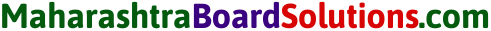Question 2.
Prove the following statements:
a. If the angle of incidence and angle of emergence of a light ray falling on a glass slab are i and e respectively, prove that i = e. (Practice Activity Sheet – 4)
In the following figure, SR || PQ and NM is the refracted ray. Hence, r = i1.
Now gna = sin i/sin r and ang = sin i1/ sin e.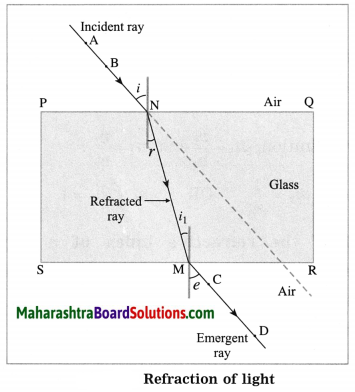Also gna = $$\frac{1}{{ }_{\mathrm{a}} n_{\mathrm{g}}}$$
∴ $$\frac{\sin i}{\sin r}=\frac{\sin e}{\sin i_{1}}$$
As r = i1, it follows that sin i = sin e
∴ i = e.

b. A rainbow is the combined effect (an exhibition) of the refraction, dispersion, and total internal reflection of light (taken together). (Practice Activity Sheet – 1)
(OR)
With a neat labelled diagram, explain how the formation of rainbow occurs.
(1) The formation of a rainbow in the sky is a combined result of refraction, dispersion, internal reflection and again refraction of sunlight by water droplets present in the atmosphere after it has rained.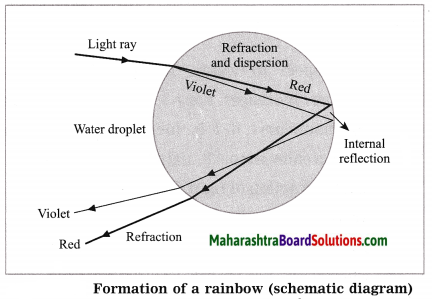Here, for simplicity only violet and red colours are shown. The remaining five colours lie between these two.

(2) The sunlight is a mixture of seven colours: violet, indigo, blue, green, yellow, orange and red. After it has stopped raining, the atmosphere contains a large number of water droplets. When sunlight is incident on a water droplet, there is (i) refraction and dispersion of light as it passes from air to water (ii) internal reflection of light inside the droplet and (iii) refraction of light as it passes from water to air.

(3) The refractive index of water is different for different colours, being maximum for violet and minimum for red. Hence, there is dispersion of light (separation into different colours) as it passes from air to water. [ See above Figure for reference.]

(4) The combined action of different water droplets, acting like tiny prisms, is to produce a rainbow with red colour at the outer side and violet colour at the inner side. The remaining five colours lie between these two.
The rainbow is seen when the sun is behind the observer and water droplets in the front.

Question 3.
Mark the correct answer in the following questions :
A. What is the reason for the twinkling of stars?
(i) Explosions occurring in stars from time to time
(ii) Absorption of light in the earth’s atmosphere
(iii) Motion of stars
(iv) Changing refractive index of the atmospheric gases
Changing refractive index of the atmospheric gases.

B. We can see the Sun even when it is little below the horizon because of
(i) reflection of light
(ii) refraction of light
(iii) dispersion of light
(iv) absorption of light
refraction of light

C. If the refractive index of glass with respect to air is 3/2, what is the refractive index of air with respect to glass?
(i) $$\frac{1}{2}$$
(ii) 3
(iii) $$\frac{1}{3}$$
(iv) $$\frac{2}{3}$$
(iv) $$\frac{2}{3}$$Question 4.
Solve the following examples:
a. If the speed of light in a medium is 1.5 × 108 m/s, what is the absolute refractive index of the medium? (Practice Activity Sheet – 1 and 4)
Solution:
Data: v = 1.5 × 108 m/s,
c = 3 × 108 m/s, n = ?
n = $$\frac{c}{v}=\frac{3 \times 10^{8} \mathrm{m} / \mathrm{s}}{1.5 \times 10^{8} \mathrm{m} / \mathrm{s}}$$ = 2
This is the absolute refractive index of the medium.

b. If the absolute refractive indices of glass and water are $$\frac{3}{2}$$ and $$\frac{4}{3}$$ respectively, what is the refractive index of glass with respect to water?
Solution: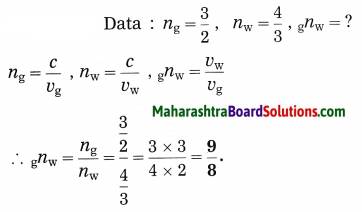This is the refractive index of glass with respect to water.

Project:

Question 1.
Using a laser and soap water. study the refraction of light under the guidance of your teacher. (Do it your self)

Can you recall? (Text Book Page No. 73)

Question 1.
What is meant by reflection of light?
Reflection of light: When light is incident on the surface of an object, in general, it is deflected in different directions. This process is called reflection of light.

Question 2.
What are the laws of reflection?
Laws of reflection of light:

1. The incident ray and the reflected ray of light are on the opposite sides of the normal to the reflecting surface at the point of incidence and all the three are in the same plane.
2. The angle of incidence j and the angle of reflection are equal in measure.

Can you recall? (Text Book page No. 75)

Question 1.
If the refractive index of the second medium with respect to the first medium is 2n1 and that of the third medium with respect to the second medium is 3n2, what and how much is 3n1.
3n1 is the refractive index of the third medium with respect to the first medium.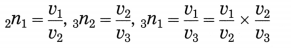3n1 = 2n1 × 3n2.

[Suppose medium 1 = air, medium 2 ≡ ice and medium 3 ≡ diamond. Then, 2n1 ÷ 1.31, 3n2 = 1.847
3n1 = 2n1 × 3n2 = 1.31 × 1.847 = 2.42 which is the refractive index of diamond with respect to air.]

Can you tell? (Textbook page No. 76)

Question 1.
Have you seen a mirage which is an illusion of the appearance of water on a hot road or in a desert?
Due to the changes in refraction of light, the light rays coming from a distant object appear to be coming from the image of the object inside the ground. This is called a mirage. When the earth’s surface is heated by the sun, the temperature of air increases. This produces a layer of hot air of lower density (mass per unit volume) and lower refractive index at the surface.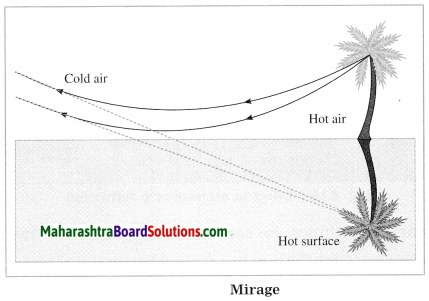Hot air works as an optically rarer medium relative to cool air. When the temperature changes rapidly in the vertical direction, as refraction of light takes place, the angle of refraction changes continuously. The rays of light from the top of an object such as a car or tree cross the rays from the bottom of the object on their way to the observer’s eye.

Hence, an inverted image is formed below the object’s true position and downward towards the surface in the direction of air at higher temperature. In this case, some rays of light bend back up into the denser air above figure. Mirage produces an impression of water near the hot ground.Question 2.
Have you seen that objects beyond and above a holy fire appear to be shaking? Why does this happen?
The temperature of air beyond and above a holy fire changes all the time. Hence, the density of air also changes constantly. Hence, the direction of propagation of the rays of light approaching us from the objects beyond and above the holy fire changes constantly. Therefore, those objects appear to be shaking.

Use your brain power! (Text Book Page No. 77)

Question 1.
From incident white light how will you obtain white emergent light by making use of two prisms?

• Take a prism. Allow white light to fall on it.
• Obtain a spectrum.
• Take a second identical prism. Place it parallel to the first prism in an upside down position with the first prism [as shown in Figure]
• Allow the colours of the spectrum to pass through the second prism.
• Obtain the beam of light emerging from the other side of the second prism.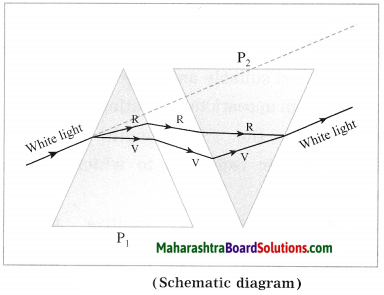The beam of light emerging from the other side of the second prism is a beam of white light.

Explanation: White light is made up of seven colours. The first prism produces dispersion of white light while the second prism combines light of different colours to produce white light again. The net deviation of a ray of light is zero.
[Note: This experiment is due to sir Isaac Newton. It proved that it was not the prism which added colours to the white light but a property of the white light itself.]

Question 2.
You must have seen chandeliers having glass prisms. The light from a tungsten bulb gets dispersed while passing through these prisms and we see coloured spectrum. If we use an LED light instead of a tungsten bulb, will we be able to see the same effect?
Light emitted by LED (light-emitting-diode) does not have all wavelengths in the region 400 nm to 700 nm. Hence, its spectrum is not the same as that of light from a tungsten bulb or as that of sunlight.

Fill in the blanks and rewrite the statements:

Question 1.
The phenomenon of change in the………..of light when it passes obliquely from one transparent medium to another is called refraction.
The phenomenon of change in the direction of propagation of light when it passes obliquely from one transparent medium to another is called refraction.

Question 2.
The refractive index depends upon the…………of propagation of light in different media.
The refractive index depends upon the velocity of propagation of light in different media.Question 3.
The process of separation of light into its component colours while passing through a medium is called………..
The process of separation of light into its component colours while passing through a medium is called dispersion of light.

Question 4.
When a light ray travels obliquely from air to water, it bends………the normal at the point of incidence.
When a light ray travels obliquely from air to water, it bends towards the normal at the point of incidence.

Question 5.
When a light ray travels obliquely from benzene to air, it bends…………the normal at the point of incidence.
When a light ray travels obliquely from benzene to air, it bends away from the normal at the point of incidence.

Question 6.
In glass, the speed of red ray is……violet ray.
In glass, the speed of red ray is greater than that of violet ray.

Question 7.
The speed of light in glass is………in water.
The speed of light in glass is less than that in water.

Question 8.
The speed of light in water is…………in benzene.
The speed of light in water is greater than that in benzene.

Question 9.
Rainbow occurs due to refraction, dispersion,……….and again refraction of sunlight by water droplets.
Rainbow occurs due to refraction, dispersion, internal reflection and again refraction of sunlight by water droplets.

Question 10.
In dispersion of sunlight by a glass prism,………..ray is deviated the least.
In dispersion of sunlight by a glass prism, red ray is deviated the least.

Rewrite the following statements by selecting the correct options:

Question 1.
The change in the direction of propagation of light when it passes obliquely from one transparent medium to another is called………
(a) dispersion
(b) scattering
(c) refraction
(d) reflection
(c) refraction

Question 2.
When a ray of light travels from air to glass slab and strikes the surface of separation at 90°, then it…………
(a) bends towards the normal
(b) bends away from the normal
(c) passes unbent
(d) passes in zigzag way
(c) passes unbentQuestion 3.
If a ray of light passes from a denser medium to a rarer medium in a straight line, the angle of incidence must be…………
(a) 0°
(b) 30°
(c) 60°
(d) 90°
(a) 0°

Question 4.
A ray of light strikes a glass slab at an angle of 50° with the normal to the surface of the slab. What is the angle of incidence?
(a) 50°
(b) 25°
(c) 40°
(d) 100°
(a) 50°

Question 5.
If a ray of light propagating in air strikes a glass slab at an angle of 60° with the surface of the slab, the angle of refraction is…………
(a) more than 30 °
(b) less than 30 °
(c) 60°
(d) 30°
(b) less than 30 °

Question 6.
A ray of light gets deviated When it passes obliquely from one medium to another medium because………..
(a) the colour of light changes
(b) the frequency of light changes
(c) the speed of light changes
(d) the intensity of light changes
(c) the speed of light changes

Question 7.
The speed of light in turpentine oil is 2 × 108 m/s. The absolute refractive index of turpentine oil is about……..[Speed of light in vacuum ≈ 3 × 108 m/s]
(a) 1.5
(b) 2
(c) 1.3
(d) 0.67
(a) 1.5

Question 8.
LASER stands for………..
(a) light amplification by stimulated emission of radiation
(b) light and sound energy radiation
(c) light and simulated energy radiation
(d) light amplification by sound energy radiation
(a) light amplification by stimulated emission of radiation

Question 9.
Out of the following……….has the highest absolute refractive index.
(a) fused quartz
(b) diamond
(c) crown glass
(d) ruby
(b) diamond

Question 10.
The absolute refractive index…………
(a) is expressed in dioptre
(b) is expressed in m/s
(c) of air is about $$\frac{4}{3}$$
(d) has no unit
(d) has no unit

Question 11.
The speed of light in a medium of refractive index n is………., where c is the speed of light
in vacuum.
(a) $$\frac{c}{n}$$
(b) nc
(c) $$\frac{n}{c}$$
(d) $$\sqrt{\frac{c}{n}}$$
(a) $$\frac{c}{n}$$

Question 12.
The speed of light in a transparent medium having absolute refractive index 1.25 is……….[Speed of light in vacuum ≈ 3 × 108 m/s]
(a) 1.25 × 108 m/s
(b) 2.4 × 108 m/s
(c) 3.0 × 108 m/s
(d) 1.5 × 108 m/s
(b) 2.4 × 108 m/sQuestion 13.
…………light is deviated the maximum in the spectrpm of white light obtained with a glass prism.
(a) Red
(b) Yellow
(c) Violet
(d) Blue
(c) Violet

Question 14.
………..light is deviated the least in the spectrum of white light obtained with a glass prism.
(a) Red
(b) Yellow
(c) Violet
(d) Blue
(a) Red

Question 15.
A ray of light makes an angle of 50° with the surface S1 of the glass slab. Its angle of incidence will be………….(March 2019)
(a) 50°
(b) 40°
(c) 140°
(d) 0°
(a) 50°

Question 16.
A glass slab is placed in the path of convergent light. The point of convergence of light:
(a) moves away from the slab
(b) moves towards the slab
(c) remains at the same point
(d) undergoes a lateral shift
(a) moves away from the slab

Question 17.
In refraction of light through a glass slab, the directions of the incident ray and the refracted ray are………… (Practice Activity Sheet – 1)
(a) perpendicular to each other
(b) non-parallel to each other
(c) parallel to each other
(d) intersecting each other
(c) parallel to each other

Question 18.
If we gradually increase the angle of incidence of a ray of light passing through a prism, then………….. (Practice Activity Sheet – 4)
(a) the angle of deviation goes on decreasing
(b) the angle of deviation decreases but after certain value of incident angle, deviation angle increases
(c) the angle of deviation goes on increasing
(d) the angle of deviation increases but after certain value of incident angle, deviation angle decreases
(b) the angle of deviation decreases but after certain value of incident angle, deviation angle increases

State whether the following statements are True or False. (If a statement is false, correct it and rewrite it.):

Question 1.
The incident ray and the refracted ray of light are on the opposite sides of the normal at the point of incidence.
True.

Question 2.
The refractive index of a medium (such as glass) does not depend on the wavelength of light.
False. (The refractive index of a medium depends on the wavelength of light.)

Question 3.
When a light ray travels obliquely from an optically rarer medium to an optically denser medium, it bends away from the normal.
False. (When a light ray travels obliquely from an optically rarer medium to an optically denser medium, it bends towards the normal.)Question 4.
When a light ray travels obliquely from glass to air, it bends towards the normal.
False. (When a light ray travels obliquely from glass to air, it bends away from the normal.)

Question 5.
If the angle of incidence is 0°, the angle of refraction is 90°.
False. (If the angle of incidence is 0°, the angle of refraction is also 0°.)

Question 6.
In dispersion of white light by a glass prism, yellow colour is deviated the least.
False. (In dispersion of white light by a glass prism, red colour is deviated the least.)

Question 7.
In vacuum, the speed of light does not depend upon the frequency of light.
True.

Question 8.
In glass, the speed of violet ray is less than that of red ray.
True.

Question 9.
In a material medium, the speed of light depends on the frequency of light.
True.

Question 10.
The velocity of light is different in different media.
True.

Question 11.
Wavelength of red light is close to 700 nm.
True.

Question 12.
Wavelength of orange light is greater than that of blue light.
True.

Find the odd one out and give the reason:

Question 1.
Reflection, Neutralization, Refraction, Dispersion.
Neutralization. It is associated with a chemical reaction between an acid and an alkali; others are phenomena associated with light.

Answer the following questions in one sentence each:

Question 1.
Mention any two phenomena in nature where refraction of light takes place.
Mirage and twinkling of a star.

Question 2.
What is the angle of refraction when the angle of incidence is 0°?
When the angle of incidence is 0°, the angle of refraction is also 0°.

Question 3.
In refraction of light, $$\frac{\sin i}{\sin r}$$ = constant in sin a particular case. What is this constant called?
The constant $$\frac{\sin i}{\sin r}$$ (in a particular case) is called the refractive index of the second medium with respect to the first medium.

Question 4.
If the refractive index of medium 2 with respect to medium 1 is 5/3, what is the refractive index of medium 1 with respect to medium 2?
The refractive index of medium 1 with respect to medium 2 is 0.6.Question 5.
In dispersion of sunlight by a glass prism, which colour is deviated the least?
In dispersion of sunlight by a glass prism, red colour is deviated the least.

Question 6.
In dispersion of sunlight by a glass prism, which colour is deviated the most?
In dispersion of sunlight by a glass prism, violet colour is deviated the most.

Question 7.
What is the wavelength of violet light?
The wavelength of violet light is (about) 400 nm.

Question 8.
State the relation between 2n1 and critical angle.
2n1 = sin i, where i is the critical angle.

Question 1.
What is meant by refraction of light?
The change in the direction of propagation of light when it passes obliquely from one transparent medium to another is called refraction of light.

Question 2.
Why is there a change in the direction of propagation of light when it passes obliquely from one transparent medium to another?
The velocity of light is different in different media. Hence, there is a change in the direction of propagation of light when it passes obliquely from one transparent medium to another.

Question 3.
In the case of refraction of light through a glass slab, the emergent ray is parallel to the incident ray, but it is displaced sideways. Why does this happen?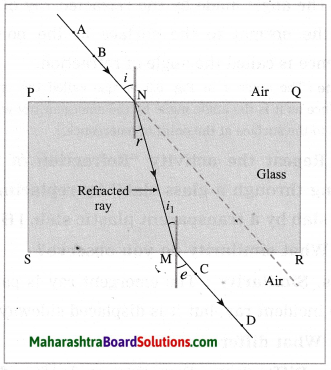The first refraction takes place as light passes obliquely from air to glass. In this case, the ray of light bends towards the normal at point N. The second refraction takes place as light passes obliquely from glass to air. In this case, the ray of light bends away from the normal at point M. The faces PQ and SR of the glass slab are parallel. Hence, the extent of bending of light at SR is equal in magnitude but opposite in sense relative to the bending of light at PQ. Hence, the emergent ray of light (MD) is parallel to the incident ray of light (AN), but it is displaced sideways as shown in Figure.

Question 4.
Define angle of incidence and angle of refraction.
(1) The angle made by the incident ray of light with the normal to the surface at the point of incidence is called the angle of incidence.

(2) The angle made by the refracted ray of light with the normal to the surface at the point of incidence is called the angle of refraction.

[Note: The angle e in Fig. 6.3 is also called the angle of emergence as it is the angle made by the emergent ray with the normal to the surface at the point of emergence. ]Question 5.
Repeat the activity “Refraction of light passing through a glass sl^b” by replacing the glass slab by a transparent plastic slab.
(i) What similarity do you observe?
(ii) What difference do you notice?
(i) Similarity: The emergent ray is parallel to the incident ray, but it is displaced sideways.
(ii) Difference: For a given angle of incidence, the extent of refraction (bending) is different (in general, less) for a transparent plastic slab relative to the glass slab.

Question 6.
State the laws of refraction of light.
Laws of refraction of light:
(1) The incident ray and the refracted ray are on the opposite sides of the normal to the surface at the point of incidence and all the three, i.e., the incident ray, the refracted ray and the normal are in the same plane.

(2) For a given pair of media, the ratio of the sine of the angle of incidence to the sine of the angle of refraction is constant (Snell’s law). This constant is called the refractive index of the second medium with respect to the first medium.
[Note: Here, a ray means a ray of light.]

Question 7.
How is refraction of light related to refractive index?
When a ray of light travels obliquely from an optically rarer medium (lower refractive index) to an optically denser medium (higher refractive index), the ray bends towards the normal. When a ray of light travels obliquely from an optically denser medium to an optically rarer medium, the ray bends away from the normal. For a given angle of incidence (i ≠ 0), the extent of refraction (bending) of light is different in different media.

If the refractive index of the second medium with respect to the first medium is greater than 1, the greater the refractive index, the greater is the bending of the ray of light towards the normal. If the refractive index of the second medium with respect to the first medium is less than 1, the greater the refractive index, the lesser is the bending of the ray of light away from the normal.

Question 8.
Define the refractive index of the second medium with respect to the first medium.
(OR)
What is meant by refractive index?
The refractive index of the second medium with respect to the first medium is defined as the ratio of the sine of the angle of incidence to the sine of the angle of refraction when the ray of light is obliquely incident at the boundary separating the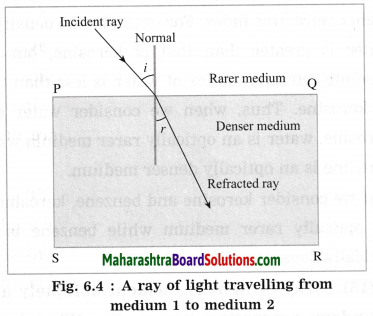two media and travels from the first medium to the second medium. (See Fig. 6.4.)
(OR)
The refractive index of the second medium with respect to the first medium is defined as the ratio of (the magnitude of) the velocity of light in the first medium to (the magnitude of) the velocity of light in the second medium.

[Note: Velocity is a vector, i.e., it has magnitude and direction. In definition of refractive index, we consider only the magnitude of velocity of light (speed of light). Velocity of light in a medium depends on the physical condition of the medium as well as the frequency of light. Velocity of light is different in different media. For a given medium, the refractive index depends on the colour of light (frequency of light.)]

Question 9.
State the formulae for the refractive index of the second medium with respect to the first medium.
The refractive index of the second medium with respect to the first medium,
2n1 = $$\frac{\sin i}{\sin r}=\frac{v_{1}}{v_{2}}$$
where i is the angle of incidence, r is the angle of refraction (as the ray of light passes obliquely from the first medium to the second medium), v1 is the magnitude of the velocity (speed) of light in the first medium and v2 is the magnitude of the velocity of light in the second medium.Question 10.
Define absolute refractive index.
The absolute refractive index of a medium is defined as the ratio of the magnitude of the velocity of light in vacuum to the magnitude of the velocity of light in the medium.

[Note: The speed of light is maximum in vacuum, about 3 × 108 m/s. When light travels from one medium to another, there occurs a change in its speed and wavelength (A). But its frequency (v) remain the same.]

Question 11.
Obtain the relation between the refractive index of the second medium with respect to the first medium and the refractive index of the first medium with respect to the second medium.
Let v1 = speed of light in the first medium, v2 = speed of light in the second medium, 2n1 = refractive index of the second medium With respect to the first medium and 1n2 = refractive index of the first medium with respect to the second medium.
By definition, 2n1 = $$\frac{v_{1}}{v_{2}}$$ and 1n2 = $$\frac{v_{2}}{v_{1}}$$
Hence,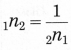(OR)
1n2 × 2n1 = 1.

Question 12.
If the refractive index of a certain material with respect to air is 1.5, what is the refractive index of air with respect to that material?
As the refractive index of the given material with respect to air is 1.5, the refractive index of air with respect to the material is
$$\frac{1}{1.5}=\frac{1}{3 / 2}=\frac{2}{3}$$ = 0.6667 (approximately)

Question 13.
Explain the terms optically rarer medium and optically denser medium with examples.
When we consider two media (such as air and glass), the medium with lower refractive index is called the optically rarer medium (in the present case, air) and the medium with higher refractive index is called the optically denser medium (glass, in the present case).

The higher density does not necessarily mean higher refractive index. For example, the density of water is greater than that of kerosene, but the absolute refractive index of water is less than that of kerosine. Thus, when we consider water and kerosine, water is an optically rarer medium while kerosine is an optically denser medium.

If we consider kerosene and benzene, kerosine is an optically rarer medium while benzene is an optically denser medium.

Question 14.
A ray of light is incident obliquely at a boundary separating two media. What is its behaviour if (1) the refractive index of the second medium is greater than that of the first medium (2) the refractive index of the first medium is greater than that of the second medium? Draw the corresponding neat and labelled diagrams.
Consider a ray of light incident obliquely at a boundary separating two media.
(1) If the refractive index of the second medium is greater than that of the first medium, the ray bends towards the normal at the point of incidence as it travels from the first medium (optically rarer medium) to the second medium (optically denser medium). The angle of refraction (r) is less than the angle of incidence (i). (Fig. 6.6)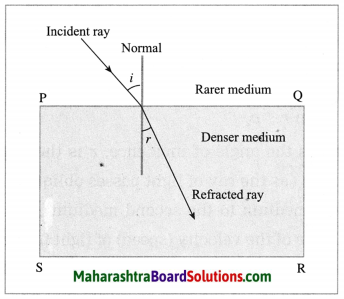Fig. 6.6: A ray of light travelling from a rarer medium to a denser medium (Schematic diagram)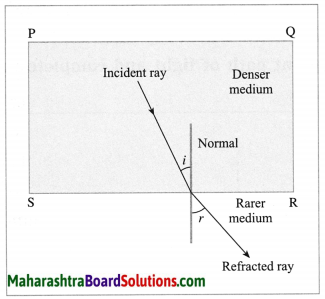Fig. 6.7: A ray of light travelling from a denser medium to a rarer medium (Schematic diagram)

(2) If the refractive index of the first medium is greater than that of the second medium, the ray bends away from the normal at the point of incidence as it travels from the first medium (optically denser medium), to the second medium (optically rarer medium). The angle of refraction (r) is greater than the angle of incidence (i). (Fig. 6.7)

[Note In this chapter, a rarer medium means an optically rarer medium and a denser medium means optically denser medium unless stated otherwise.]Question 15.
Observe the following figure and write accurate conclusion regarding refraction of light. (Practice Activity Sheet – 2)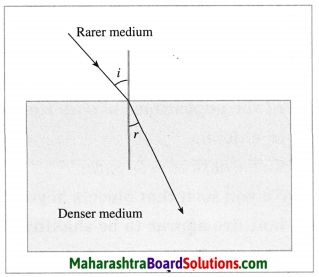When a light ray passes obliquely from a rarer medium to a denser medium, it bends towards the normal.

Question 16.
What happens when a ray of light is incident normal to the interface between two media? Draw the corresponding neat and labelled diagram.
When a ray of light is incident normal to the interface between two media, the ray propagates undeviated as it travels from the first medium to the second medium irrespective of the refractive indices of the two media. In this case, the angle of incidence (i) is zero and so also the angle of refraction (r).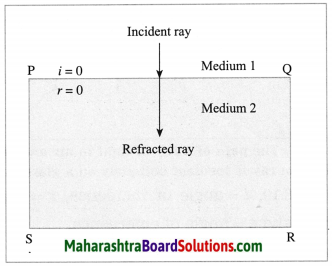Fig. 6.9: A ray of light incident normal to the interface between two media propagates without any change in its direction of propagation

Question 17.
Draw a neat and labelled diagram to show the path of a ray of light in air and glass when the ray is incident obliquely on a glass slab. Show the (i) incident ray (ii) refracted ray (iii) emergent ray (iv) angle of incidence (v) angle of refraction (vi) angle of emergence in the diagram.
(OR)
Draw a neat and labelled diagram to show refraction of light through a glass slab.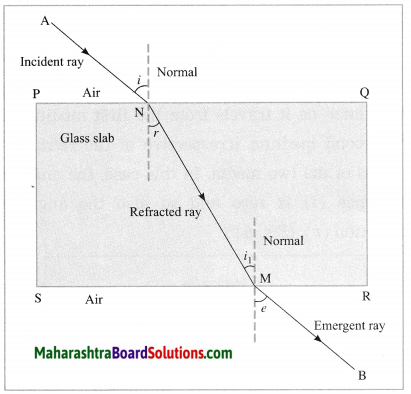Fig. 6.10: The path of the ray of light in air and glass when the ray is incident obliquely on a glass slab
In Fig. 6.10, i = angle of incidence, r = angle of refraction and e = angle of emergence.

Question 18.
Observe the given figure and name the following rays: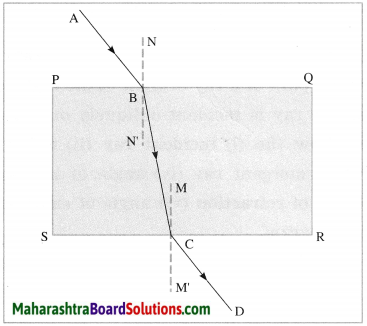(i) ray AB
(ii) Ray BC
(iii) ray CD
(i) The ray AB is the incident ray.
(ii) The ray BC is the refracted ray.
(iii) The ray CD is the emergent ray.Question 19.
A plane mirror is kept at the bottom of a trough with water in it as shown in the following figure (Fig. 6.12). The ray of light emerging from a source at the point S outside the trough reaches the point A on the surface of water. Draw a neat ray diagram to show the subsequent path of light and complete the ray diagram.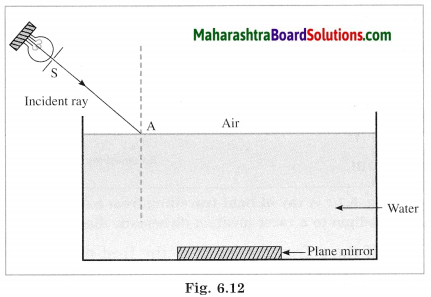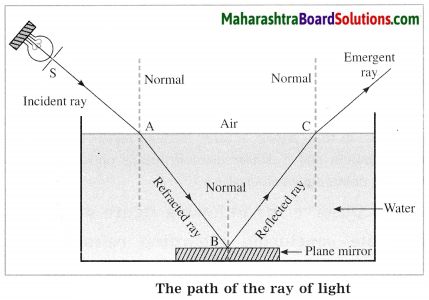Question 20.
Give two examples of the effect of atmospheric refraction on a small scale in local environment.

1. The occurrence of a mirage
2. Flickering of an object seen through a turbulent stream of hot air rising above the Holi fire are examples of the effect of atmospheric refraction on a small scale in local environment.

Question 21.
What is a mirage? With a neat labelled diagram, explain the conditions under which it is seen.
Due to the changes in refraction of light, the light rays coming from a distant object appear to be coming from the image of the object inside the ground. This is called a mirage.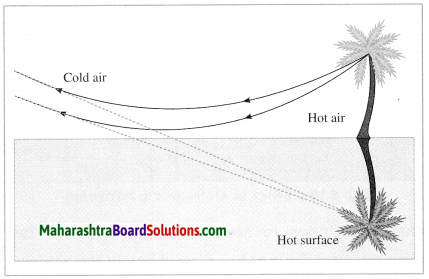When the earth’s surface is heated by the sun, the temperature of air increases. This produces a layer of hot air of lower density (mass per unit volume) and lower refractive index at the surface. Hot air works as an optically rarer medium relative to cool air. When the temperature changes rapidly in the vertical direction, as refraction of light takes place, the angle of refraction changes continuously.

The rays of light from the top of an object such as a car or tree cross the rays from the bottom of the object on their way to the observer’s eye. Hence, an inverted image is formed below the object’s true position and downward towards the surface in the direction of air at higher temperature. In this case, some rays of light bend back up into the denser air above figure. Mirage produces an impression of water near the hot ground.

Question 22.
Explain in brief the flickering of an object seen through a turbulent stream of hot air rising above the Holi fire.
During the Holi fire, the temperature of the air just above the fire becomes much greater than that of the air further up. The hot air has lower density (mass per unit volume) and lower refractive index. It becomes an optically rarer medium. The cool air has higher density and higher refractive index. It is an optically denser medium relative to hot air. Hence, in refraction of light, the angle of refraction changes continuously due to a continuous variation in refractive index.

As the physical conditions of air change rapidly, the apparent position of an object fluctuates rapidly. This gives rise to the flickering of an object seen through a turbulent stream of hot air rising above the Holi fire.Question 23.
With a neat labelled diagram, explain twinkling of a star. Also explain why a planet does not twinkle.
(1) As a star is far away from the earth, it appears as a point source of light. The density of air decreases with height above the earth’s surface. Hence, the refractive index of air also decreases with height. When starlight enters the earth’s atmosphere, it undergoes refraction continuously in the medium with gradually changing refractive index. The bending of starlight occurs towards the normal as it passes from the optically rarer part of the medium to the optically denser part.

(2) Hence, when a star is observed near the horizon, its apparent position is slightly higher than the actual position (See below figure).

(3) Further, the apparent position varies with time as the medium is not stationary due to mobility of air and change in temperature. When more light is refracted towards the observer the star appears bright. When less light is refracted towards the observer, the star appears dim.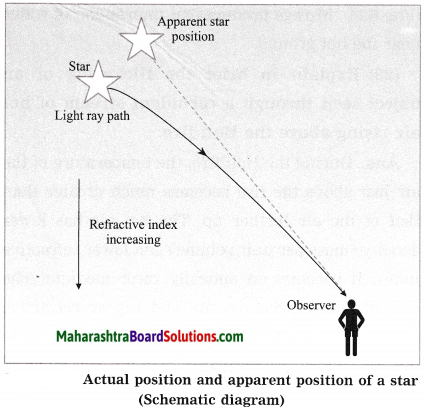(4) Thus there is fluctuation in the brightness of a star when observed from the earth. This is called twinkling of a star.

(5) Compared to stars, planets are relatively closer to the earth. Hence, a planet appears as a collection of a large number of point sources. Due to the changes in the refractive index of air, there is a change in the position and brightness of these point sources.

There is an increase in intensity of light coming from some point sources while there is a decrease in intensity of light coming from equal number of other point sources, on an average. The average brightness of a planet remain the same. Also, there is no change in the average position of a star. Hence, a planet does not twinkle.

Question 24.
What is the correct reason for blinking/flickering of stars? Explain it.
(a) The blasts in the stars.
(b) Absorption of star light by the atmosphere.
(c) Motion of the stars.
(d) Changing refractive index of gases in the atmosphere. (Practice Activity Sheet – 2)
(d) Changing refractive index of the gases in the atmosphere results in blinking/flickering of stars.

Explanation:
(1) As a star is far away from the earth, it appears as a point source of light. The density of air decreases with height above the earth’s surface. Hence, the refractive index of air also decreases with height. When starlight enters the earth’s atmosphere, it undergoes refraction continuously in the medium with gradually changing refractive index. The bending of starlight occurs towards the normal as it passes from the optically rarer part of the medium to the optically denser part.

(2) Hence, when a star is observed near the horizon, its apparent position is slightly higher than the actual position (See below figure).

(3) Further, the apparent position varies with time as the medium is not stationary due to mobility of air and change in temperature. When more light is refracted towards the observer the star appears bright. When less light is refracted towards the observer, the star appears dim.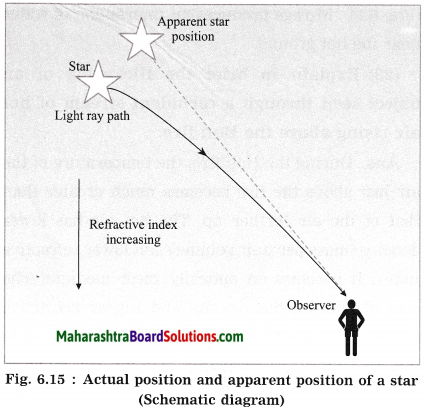(4) Thus there is fluctuation in the brightness of a star when observed from the earth. This is called twinkling of a star.

(5) Compared to stars, planets are relatively closer to the earth. Hence, a planet appears as a collection of a large number of point sources. Due to the changes in the refractive index of air, there is a change in the position and brightness of these point sources.

There is an increase in intensity of light coming from some point sources while there is a decrease in intensity of light coming from equal number of other point sources, on an average. The average brightness of a planet remain the same. Also, there is no change in the average position of a star. Hence, a planet does not twinkle.

Question 25.
With a neat labelled diagram, explain advanced sunrise and delayed sunset.
(1) The sunrise (the appearance of the sun above the horizon) is advanced due to atmospheric refraction of sunlight. An observer on the earth sees the sun two minutes before the sun reaches the horizon. A ray of sunlight entering the earth’s atmosphere follows a curved path due to atmospheric refraction before reaching the earth. This happens due to a gradual variation in the refractive index of the atmosphere.

For the observer on the earth, the apparent position of the sun is slightly higher than the actual position. Hence, the sun is seen before the sun reaches the horizon.

(2) Increased atmospheric refraction of sunlight occurs also at the sunset (the sun disappearing below the horizon). In this case, the observer on the earth continues to see the setting sun for two minutes after the sun has dipped below the horizon, thus delaying the sunset.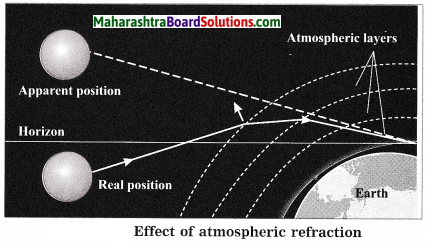The advanced sunrise and delayed sunset increases the duration of day by four minutes.Question 26.
Water in a swimming pool or water tank appears shallower than its depth. Why?
When light rays travel obliquely from an optically denser medium (water, in this case) to an optically rarer medium (air, in this case), they bend away from the normal at the point of incidence. As a result, the bottom of a swimming pool or water tank appears raised to an observer standing near the edge of the pool or the tank. Therefore, the swimming pool or water tank appears shallower than its depth.

Question 27.
Place a coin at the bottom of a glass jar containing water. Now tilt the jar suitably. When viewed at a suitable angle, the coin appears to be floating. Why?
When light rays travel obliquely from an optically denser medium (water, in this case) to an optically rarer medium (air, in this case), they bend away from the normal at the point of incidence. As a result, the coin appears raised. Therefore, when the jar is tilted suitably and observed at a suitable angle, the coin appears to be floating.

Question 28.
State the wavelength range of electromagnetic radiation to which our eyes are sensitive.
Our eyes are sensitive to light (electromagnetic radiation). Its wavelength range is 400 nm to 700 nm.
[Note: Wavelength (λ) goes on decreasing and frequency (ν) goes on increasing from red (λ ≃ 700 nm) → orange → yellow → green → blue → indigo → violet (A ≃ 400 nm). c = vλ, where c is the speed of light in vacuum.]

Question 29.
What do you mean by dispersion of light? What is a spectrum of light? Name the different colours of light in the proper sequence in the spectrum of white light.
(OR)
What do you mean by dispersion? Name the different colours of light in the proper sequence in the spectrum of white light.
The process of separation of light into its component colours while passing through a medium is called dispersion of light. The band of coloured components of a light beam is called spectrum.
The different colours of light in the spectrum of white light are violet, indigo, blue, green, yellow, orange and red.

Question 30.
What is a prism?
A prism is a transparent medium bound by two plane surfaces inclined at an angle. Normally it is made of glass and has triangular cross section.

Question 31.
With a neat labelled diagram, describe the experiment to demonstrate dispersion of sunlight (white light) by a prism.
Experiment:
(1) Procedure: Keep a glass prism on a table in a dark room. Hold a plane mirror outside the room so that it reflects a beam of sunlight into the room. Allow this beam to pass through a narrow slit made in cardboard and then fall on the prism. Place a white screen on the other side of the prism as shown in the following figure. [Fig. 6.17]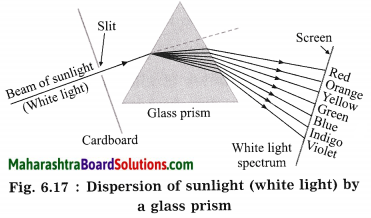(2) Observations:

1. A pattern of various colours is observed on the screen. This pattern is called the spectrum.
2. It is found that in dispersion, the ray corresponding to violet colour deviates the most.
3. The ray corresponding to red colour deviates the least.
4. The deviation of rays corresponding to other colours is intermediate.

(3) Conclusion: When sunlight (white light) is incident on a prism, dispersion of light takes place, forming a spectrum.

[Notes: (1) This experiment is due to Sir Isaac Newton (1642 – 1727), English physicist and mathematician. (2) If in a Board examination, incomplete diagram (as shown in Fig. 6.18) is given, students should complete it and label its parts as shown in Fig. 6.17.]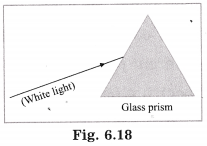Question 32.
How does the dispersion of white light take place when it passes through a glass prism?
When rays of light are incident on a prism, they are refracted twice, while travelling from air to glass and then from glass to air. Even when the incident rays are directed away from the base of the prism, the emergent rays bend towards the base of the prism, as the prism is triangular. Thus, the rays are deviated as they pass through the prism.

The refractive index of glass is different for different colours. Therefore, the rays corresponding to different colours are deviated to different extents. White light is a mixture of seven colours : violet, indigo, blue, green, yellow, orange and red. Hence, when white light is incident on a prism, a spectrum of seven colours is obtained.

The refractive index of glass is maximum for violet light and minimum for red light. Hence, violet light is deviated the most and red light is deviated the least. The deviation of rays corresponding to other colours is intermediate. In this manner, the dispersion of light takes place when it passes through a glass prism. [For reference, see Fig. 6.17.]

Question 33.
What is a spectrum? Why do we get a spectrum of seven colours when while light is dispersed by a prism?
(OR)
Explain how a spectrum is formed.
A band of coloured components of a light beam is called a spectrum. When white light is incident on a prism, the rays corresponding to different colours bend through different angles on refraction.

Of the various colours in the visible region, red light bends the least and violet light bends the most. Each colour emerges through the prism along a different path and becomes, distinct. Hence, we get a spectrum of seven colours.

Question 34.
What is partial reflection of light?
When light travels from a denser medium to a rarer medium, it is partially reflected, i.e., part of light comes back into the denser medium as per the laws of reflection. This is called partial reflection of light.

[Note: Partial reflection of light occurs even when light travels from a rarer medium to a denser medium. The rest of light is refracted.]

Question 35.
Explain the terms total internal reflection and critical angle.
Figure 6.20 shows passage of light from water (denser medium) to air (rarer medium).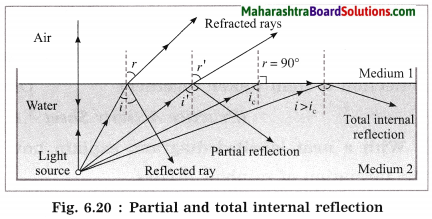The ray of light incident at the boundary separating the two media bends away from the normal on refraction. Here, the angle of refraction r, is greater than the angle of incidence i.
Now anw = $$\frac{\sin i}{\sin r}$$ < 1. Here, anw is the refractive index of sin r air with respect to water.

As anw is constant, r increases as i increases. For r = 90°, the ray travels along the boundary. If i is increased further, as r cannot be greater than 90°, light does not enter air. There is no refraction of light and all the light enters water on reflection. This is called total internal reflection.
For r = 90°, anw = $$\frac{\sin i}{\sin 90^{\circ}}$$ = sin i. This angle i is sin 90° called the critical angle.

Question 36.
Swarali has got the following observations while doing an experiment. Answer her questions with the help of observations. (Practice Activity Sheet – 2)
Swarali observed that the light bent away from the normal, while travelling from a denser medium to a rarer medium. When Swarali increased the values of the angle of incidence (i). the values of the angle of refraction (r) went on increasing. But at a certain angle of incidence, the light rays returned into the denser medium.
So, Swarali has some questions. Answer them.
(a) Name this certain value of i. What is the value of r at that time?
(b) Name this process of returning light in the denser medium. Explain the process.
(a) Critical angle r = 90°
(b) Total internal reflection.

As light goes from a denser to rarer medium, if the value of the angle of incidence increases, then the value of the angle of refraction also increases. But after a specific angle of incidence called the critical angle, the light gets reflected back into the denser medium.

The ray of light incident at the boundary separating the two media bends away from the normal on refraction. Here, the angle of refraction r, is greater than the angle of incidence i.
Now anw = $$\frac{\sin i}{\sin r}$$ < 1. Here, anw is the refractive index of sin r air with respect to water. As anw is constant, r increases as i increases. For r = 90°, the ray travels along the boundary. If i is increased further, as r cannot be greater than 90°, light does not enter air. There is no refraction of light and all the light enters water on reflection. This is called total internal reflection.

For r = 90°, anw = $$\frac{\sin i}{\sin 90^{\circ}}$$ = sin i. This angle i is sin 90° called the critical angle.Question 37.
The observations made by Swarali while doing the experiment are given below. Based on these write answers to the questions:
Swarali found that the light ray travelling from the denser medium to a rarer medium goes away from the normal. If the angle of incidence (i) is raised by Swarali, the angle of refraction (r) went on increasing. However, after certain value of the angle of incidence, the light ray is seen to return back into the denser medium. (March 2019)
(i) What is the specific value of ∠i called?
(ii) What is the process of reflection of incident ray into a denser medium called?
(iii) Draw the diagrams of three observations made by Swarali.
(i) Critical angle
(ii) Total internal reflection
(iii)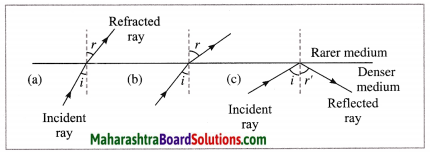Question 38.
Define total internal reflection of light.
When light travels from a denser medium to a rarer medium, if the angle of incidence is greater than the critical angle, there is no refraction of light and all the light is reflected in the denser medium. This is called total internal reflection of light.

Question 39.
Define critical angle.
When light travels from a denser medium to a rarer medium, the angle of incidence for which the angle of refraction becomes 90°, is called the critical angle.

Question 40.
If the refractive index of a rarer medium with respect to a denser medium is 0.5, what is the critical angle?
2n1 = 0.5 = sin i
∴ Critical angle i = 30°.

Question 41.
Name the devices in which total internal reflection of light is used.

1. Total internal reflecting prisms are used in a camera, binoculars, periscope.
2. Total internal reflection of light is used in optical fibres.

[Note: Total internal reflection of light plays an important role in sparkling brilliance of a diamond.]

Question 42.
Explain why an empty test tube held obliquely in water appears shiny to an observer looking down.
When an empty test tube is held obliquely in Water in a beaker, some light rays passing from water to air are incident at an angle greater than the critical angle. They are, thus, totally internally reflected as shown, and the surface of the test tube has a silvery shine.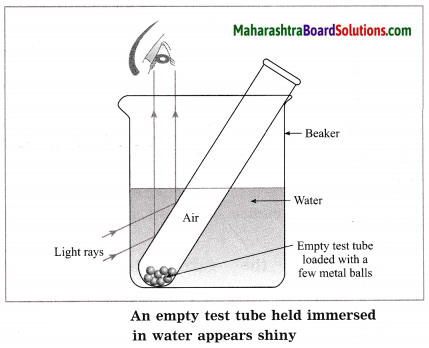Question 43.
Observe the given figure and answer the following questions. (Practice Activity Sheet – 3)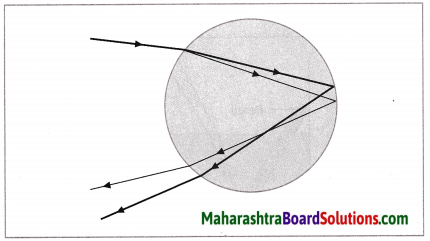(a) Identify and write the natural process shown in the figure.
(b) List the phenomena which are observed in this process.
(c) Redraw the diagram and show the above phenomena in it.
(a) The natural process shown in the figure is formation of rainbow.
(b) The phenomena observed in this process are refraction, internal reflection and dispersion of light.
(c)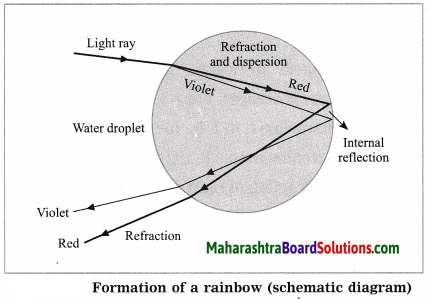Write a short note on the following:

Question 1.
Refraction observed in the atmosphere.
When a ray of light passes obliquely from an optically rarer medium to an optically denser medium, it bends towards the normal at the point of incidence. If opposite is the case, the ray bends away from the normal.

Atmosphere is never static. Air is mobile and its density and temperature are not uniform. As a result, in general, the path of a ray of light through atmosphere of varying refractive index is a curve. The refractive index of cool air is greater than that of hot air.

Atmospheric refraction of light results in many interesting optical phenomena such as twinkling of a star, advanced sunrise and delayed sunset, mirage and flickering of an object seen through a turbulent stream of hot air rising from a fire.

Question 2.
Dispersion of light.
The process of separation of light into its component colours while passing through a medium is called dispersion of light. When white light passes through a glass prism, it spreads out into a band of different colours (components) called the spectrum of light. The colours in the spectrum of white light are violet, indigo, blue, green, yellow, orange and red.

Formation of a rainbow is an example of dispersion of light in nature. In this case, raindrops are responsible for dispersion of sunlight.

Dispersion takes place because the refractive index of a material such as glass or water, is different for different colours. It is maximum for violet colour and minimum for red colour. Hence, in the spectrum of white light (sunlight) obtained with a prism, violet light is deviated the most while red light is deviated the least. The deviation of light corresponding to other colours lies in between.

Give scientific reasons:

Question 1.
A coin kept in a bowl is not visible when seen from one side. But, when water is poured in the bowl, the coin becomes visible.
(1) When the bowl is empty, the rays of light coming from the coin are obstructed by the side of the bowl, and hence the coin is not visible when seen from one side of the bowl.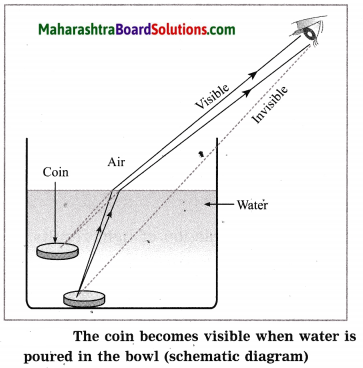(2) When water is poured in the bowl, the rays of light coming from the coin travel from water (denser medium) to air (rarer medium). Hence, they bend away from the normal on refraction. Therefore, the coin appears to be raised and becomes visible when observed from one side of the bowl.Question 2.
A pencil dipped in water obliquely appears bent at the surface of water.
(OR)
When a pencil is partly immersed in water and held in a slanting position, it appears to be bent at the boundary separating water and air.
(1) When a pencil is partly immersed in water and held in a slanting position, the rays of light coming from the immersed part of the pencil emerge from water (a denser medium) and enter air (a rarer medium). During this propagation, they bend away from the normal on refraction.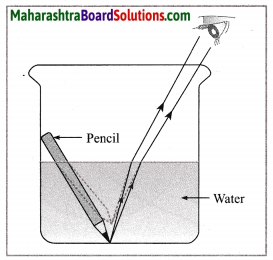The pencil appearing bent at the boundary of water and air (schematic diagram)

(2) As a result, the immersed part of the pencil does not appear straight with respect to the part outside the water, but appears to be raised. Hence, a pencil dipped obliquely in water appears bent at the surface of the water.

Question 3.
The shadow of the edge of an empty vessel is formed due to the slanting rays of the sun. When water is poured in the vessel, the shadow is shifted.
(1) When the slanting rays of the sun are obstructed by the edge of the empty vessel, the shadow of the edge is formed.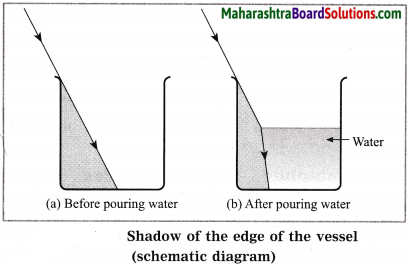(2) When water is poured in the vessel, the slanting rays of the sun travel from air (rarer medium) to water (denser medium). During this propagation, they bend towards the normal on refraction. Hence, some part in the region of the shadow is now illuminated and the shadow appears to have shifted.

Question 4.
The bottom of a pond appears raised.
(1) The rays of light coming from the bottom of a pond bend away from the normal as they travel from water (denser medium) to air (rarer medium).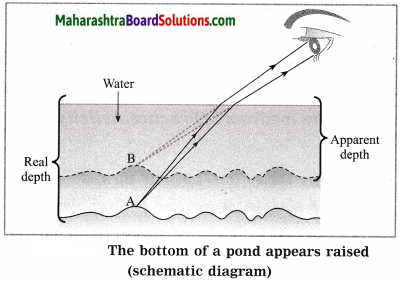(2) Hence, they appear to come from a point above the actual point from which they come.
Therefore, the bottom of the pond appears raised.

Question 5.
While shooting a fish in a lake, the gun is aimed below the apparent position of the fish.
(1) The rays of light coming from the fish bend away from the normal as they travel from water (denser medium) to air (rarer medium).
(2) Hence, the position of the fish in water appears to be above Its real position. Therefore, while shooting a fish in a lake, the gun is aimed below the apparent position of the fish.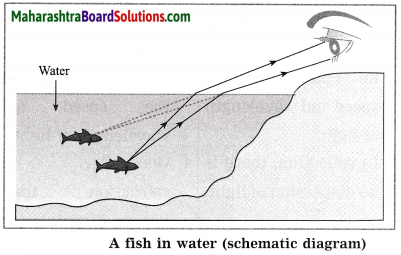Question 6.
The sun is seen on the horizon a little before sunrise.
(OR)
The sun is seen on the horizon for sometime even after sunset.
(1) The earth is surrounded by an atmosphere which is denser near the surface of the earth. When the rays of light from the sun enter the earth’s atmosphere from outer space, they travel from a rarer medium to a denser medium. Hence, they bend towards the normal on refraction.

(2) Hence, even when the sun is below the horizon while rising or setting, its rays reach us due to refraction and it appears to be on the horizon. Therefore, the sun is seen on the horizon a little before sunrise as well as for some time even after sunset.

Distinguish between:

Question 1.
Reflection of light and Refraction of light:

 Reflection of light Refraction of light 1. The rays of light, before and after reflection, travel in the same medium. 1. In refraction of light, the rays travel from one medium to another medium. 2. In reflection, the angle of incidence and the angle of reflection are equal. 2. In refraction, when the rays travel obliquely from one medium to another medium, the angle of incidence and the angle of refraction are not equal. 3. In reflection, there is no change in the speed and wavelength of light. 3. In refraction, there occurs a change in the speed and wavelength of light. 4. In reflection, there is no dispersion of light. 4. Generally, in refraction, there occurs dispersion of light.

[Note: The frequency of light remains the same in reflection and refraction.]

Complete the following or Solve and fill in the blanks :

Question 1.

 Speed of light in the first medium (v1) Speed of light in the second medium (v2) Refractive index 2n1 Refractive index 2n1 3 × 108 m/s 1.2 × 108 m/s ————————– ————————– ————————– 2.25 × 108 m/s 4/3 ————————– 2 × 108 m/s ————————– ————————– 1.5

 Speed of light in the first medium (v1) Speed of light in the second medium (v2) Refractive index 2n1 Refractive index 2n1 3 × 108 m/s 1.2 × 108 m/s 2.5 0.4 3 × 108 m/s 2.25 × 108 m/s 4/3 0.75 2 × 108 m/s 3 × 108 m/s 2/3 1.5

Formulae:
2n1 = v1/v2, 1n2 = v2/v1

Solve the following examples/numerical problems:
c = 3 × 108 m/s

Problem 1.
The speed of light in a transparent medium is 2.4 × 108 m/s. Calculate the absolute refractive index of the medium.
Solution:
Data: c = 3 × 108 m/s,
v = 2.4 × 108 m/s, n = ?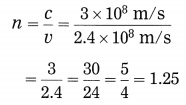The absolute refractive index of the medium = 1.25.Problem 2.
The velocity of light in a medium is 2 × 108 m/s. What is the refractive index of the medium with respect to air, if the velocity of light in air is 3 × 108 m/s?
Solution:
Data: v1 = 3 × 108 m/s,
v2 = 2 × 108 m/s, 2n1 = ?
2n1 = $$\frac{v_{1}}{v_{2}}$$
$$=\frac{3 \times 10^{8}}{2 \times 10^{8}}$$
= 1.5
The refractive index of the medium with respect to air is 1.5.

Problem 3.
Light travels with a velocity 1.5 × 108 m/s in a medium. On entering second medium its velocity becomes 0.75 × 108 m/s. What is the refractive index of the second medium with respect to the first medium? (Practice Activity Sheet – 3)
Solution:
Given: Velocity of light in the first medium = v1 = 1.5 × 108 m/s,
velocity of light in the second medium = v2 = 0.75 × 108 m/s,
refractive index of the second medium with respect to the first medium = 2n1 = ?
2n1 = $$\frac{v_{1}}{v_{2}}$$
2n1 = $$\frac{1.5 \times 10^{8}}{0.75 \times 10^{8}}$$ = 2
Hence, the refractive index of the second medium with respect to the first medium is 2.
[Note : The absolute refractive index of the second medium = $$\frac{3 \times 10^{8} \mathrm{m} / \mathrm{s}}{0.75 \times 10^{8} \mathrm{m} / \mathrm{s}}$$ = 4 (greater than that of diamond, not likely).]

Problem 4.
The refractive index of water is 4/3 and the speed of light in air is 3 × 108 m/s. Find the speed of light in water.
Solution:
Data: 2n1 = 4/3, v1 = 3 × 108 m/s, v2 = ?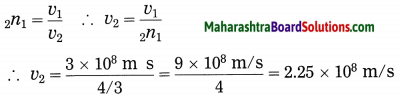The speed of light in water = 2.25 × 108 m/s.

Problem 5.
The speed of light in water and glass is 2.2 × 108 m/s and 2 × 108 m/s respectively. What is the refractive index of (i) water with respect to glass (ii) glass with respect to water?
Solution:
Data: uw = 2.2 × 108 m/s,
vg= 2 × 108 m/s, wng = ?, gnw = ?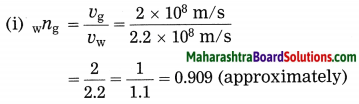The refractive index of water with respect to glass = 0.909 (approximately).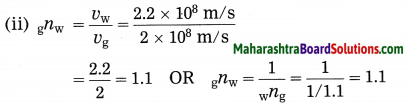The refractive index of glass with respect to glass = 1.1 (approximately).

Numerical Problems For Practice:
(Given: C = 3 × 108m/s)

Problem 1.
The speed of light in a transparent medium is 2 × 108 m/s. Find the absolute refractive index of the medium.
Solution:
1.5

Problem 2
The absolute refractive index of a transparent medium is 5/3. Find the speed of light in the medium.
Solution:
1.8 × 108 m/s

Problem 3.
The absolute refractive index of a transparent medium is 2.4 and the speed of light in that medium is 1.25 × 108 m/s. Find the speed of light in air.
Solution:
3 × 108 m/s

Problem 4.
The speed of light in water is 2.25 × 108 m/s and that in glass is 2 × 108 m/s. Find the refractive index of (i) the glass with respect to water (ii) water with respect to the glass.
Solution:
(i) 1.125
(ii) 0.889 (approximately)Problem 5.
If the refractive index of a certain glass with respect to water is 1.25, find the refractive index of water with respect to the glass.
Solution:
0.8

Problem 6.
If the absolute refractive index of glass is 1.5 and that of water is $$\frac{4}{3}$$, find the refractive index of water with respect to glass.
Solution:
$$\frac{8}{9}$$